Algebraic General Topology. Vol 1: Paperback / E-book || Axiomatic Theory of Formulas: Paperback / E-book

As an Amazon Associate I earn from qualifying purchases.

This is a draft which I will never publish. It is because this theory is unnecessarity comlex but has
no applications.
Qu asi-cartesian functions
Above we have deﬁned several diﬀerent kinds of product. These products resemble c artesian pro-
duct. Saying thi s formally, the se functions are quasi-cartesian as deﬁned below.
First (befor e formal deﬁnitions) I will give an example of a quasi-cartesian function. The ﬁrst and
the most prominent example is certain quasi-cartesian situation S together with the well known
quasi-cartesian function cartesian product of an indexed family of set s. Below is a quasi-cartesian
situation S:
1. Forms are small sets.
2. Arguments are pairs (; r) where is a form and r P.
3. The form corresponding to an argument (; r ) is .
4. Zero for the form is deﬁned by the formula Z = (; ).
The quasi-cartesian function f from S to S is deﬁned by the formula
f (λi D: (
i
; r
i
)) =
Y
i
;
Y
r
i
.
Now procee d to the formal deﬁ nitions:
Deﬁnition 1. A quasi-cartesian situation S is:
1. a set F (forms);
2. a set X (arguments);
3. a function ρ F
X
(forms of arguments);
4. a function Z X
F
(zeros)
such that ρ Z ρ = ρ.
Deﬁnition 2. The set ZC is the set of such small in dexed families of arguments that for every
small indexed family x of arguments
x ZC i dom x: x
i
= Z(ρ(x
i
)). (1)
Remark 3. For theorems below we will need only that ZC is a set of indexed families of arguments.
The formula (1) is not required, but there are no need to generalize here.
Let ﬁx two quas i-cartesian situations S
0
(source) and S
1
(destination).
Deﬁnition 4. A pre-quasi-cartesian function is a function f such that the image of f is a subset
of X
1
and every element of the domain of f is an indexed family of elements of X
0
such that:
1. x ZC
0
fx = Z
1
(ρ
1
fx) for every x dom f;
2. ρ
0
x = ρ
0
y ρ
1
fx = ρ
1
fy for every x, y dom f.
1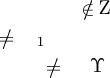There exists a function Υ (aggregation) conforming to the formula Υ(ρ
0
x) = ρ
1
fx.
A pre-quasi-cartesian function can be described ﬁr st deﬁning a function Υ from small indexed
families of forms into forms such that ρ
1
fx = Υ(ρ
0
x) and x ZC
0
fx = Z
1
Υ(ρ
0
x).
Deﬁnition 5. A quasi-cartesian function is such pre-quasi-cartesian function f that
f |
{xX
0
dom A
| ρ
0
x=A}\ZC
0
is an injection for every indexed family A of forms.
Deﬁnition 6. A pre-quasi -cartesian function with inject ive aggregation is a pre-quasi-cartesian
function for which the Υ function is injective.
Exercise 1. Prove that the above dened cartesian product of an indexed family of sets” is a quasi-cartesian
function for two quasi-cartesian systems with injective aggregation.
Deﬁnition 7 . Restriction of a quasi-cartesian situation is this quasi-cartesian si tuation with the
set of argument s X replaced by a smaller set X
such that im Z X
and forms of argumen ts ρ
replaced with ρ
= ρ|
X
.
Proposition 8. Every restriction of a quasi-cartesian situation is a quasi-cartesian situation.
Proof. We need to prove ρ
Z ρ
= ρ
. This formula follows from ρ
= ρ|
X
and dom ρ
im Z.
Deﬁnition 9 . Restriction of a pre-quasi-cartesian function is the restriction of the source quasi-
cartesian situation, the destination quasi-cartesian situation, together w ith a restriction of the
quasi-cartesian function to indexed families of the new set of (source) arguments.
Obvious 10. Restriction of a pre-quasi-cartesian function is a pre-quasi-cartesian function.
Obvious 11. Restriction of a quasi-cartesian function is a quasi-cartesian fun ction.
Obvious 12. Restriction of a (pre-)quasi- cartesian situation with injective aggregation is a (pre-
)quasi-cartesian situation with injective aggregation.
When cardhf i{x}= 1 for a binary relat ion f , we will denote f (x) or fx the element of the singleton
hf i{x}.
Proposition 13. For pre-quasi-cartesian function f we have
(hf i{x X
0
dom A
| ρ
0
x = A}) \ {Z
1
f
A)} = hf i({x X
0
dom A
| ρ x = A} \ ZC
1
).
Proof.
hf i({x X
0
dom A
| ρ
0
x = A} \ ZC
0
) =
hf i{x X
0
dom A
| ρ
0
x = A x
ZC
0
} =
hf i{x X
0
dom A
| ρ
0
x = A fx
Z
1
Υ
f
(ρ
0
x)} =
hf i{x X
0
dom A
| ρ
0
x = A fx
Z
1
Υ
f
A} =
(hf i{x X
0
dom A
| ρ
0
x = A}) \ {Z
1
f
A)}.
Proposition 14. card hf
1
i{y } = 1 if y (hf i{x X
0
dom A
| ρ
0
x = A}) \ {Z
1
f
A)}.
2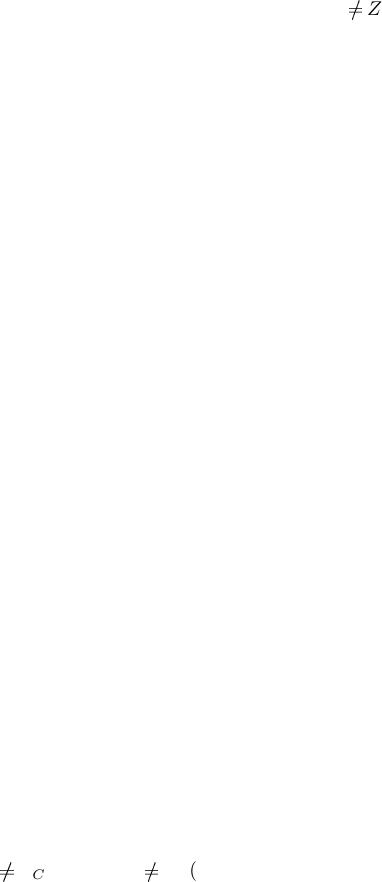Proof. card hf
1
i{y } > 1 is obvious. It remains to show that y [f
1
] a y [f
1
] b a = b for every
a and b. Really, let y [f
1
] a y [f
1
] b. Then y = fa and y = fb and thus a = b because
y hf i({x X
0
dom A
| ρ
0
x = A} \ ZC
0
) and f |
{xX
0
dom A
| ρ
0
x=A}\ZC
0
is an injection.
Fix quasi-cartesian situations S
A
, S
B
, S
C
and quasi-cartesian functions f : S
A
S
B
and g:
S
A
S
C
such that dom f = dom g. Let A is a small indexed family of forms.
[TODO: Check below
formulations (it is pos sible that I’ve done little errors confusing A, B, and C).]
For a s mall indexed family A of forms let:
ϕ
A
= g id
{xX
A
dom A
| ρ
A
x=A}
f
1
.
Obvious 15. ϕ
A
= g|
{xX
A
dom A
| ρ
A
x=A}
f
1
= g
f |
{xX
A
dom A
| ρ
A
x=A}
1
.
Proposition 16. ϕ
A
is a function and dom ϕ
A
= hf i{x X
A
dom A
| ρ
A
x = A} and for every
y do m ϕ
A
we have
ϕ
A
y =
(
Z
C
g
A) if y = Z
B
f
A);
g f
1
y if y
Z
B
f
A).
Proof. It follows from the previous proposition.
Theorem 17. ϕ
A
= g f
1
|
hf i{xX
A
dom A
| ρ
A
x=A}
.
Proof. If y (hf i{x X
A
dom A
| ρ
A
x = A}) \ {Z
B
f
A)} then card hf
1
i{y } = 1 and thus
hf i{y } {x X
B
dom A
| ρ
B
x = A} \ ZC
B
. Cons equently
hϕ
A
i{y } = hg f
1
i{y } =
g f
1
|
hf i{xX
A
dom A
| ρ
A
x=A}
{y}.
hϕ
A
i{Z
B
f
A)} = Z
C
g
A) and
g f
1
|
hf i{xX
A
dom A
| ρ
A
x=A}
{Z
C
g
A)} =
hg f
1
i{Z
C
g
A)} =
hgi({x X
A
dom A
| ρ
A
x = A} ZC
A
) =
Z
C
g
A).
Thus hϕ
A
i{y } =
g f
1
|
hf i{xX
A
dom A
| ρ
A
x=A}
{y} for every y hf i{x X
A
dom A
| ρ
A
x = A}.
Theorem 18. ϕ
A
is a bijection
hf i{x X
A
dom A
| ρ
A
x = A} hg i{x X
A
dom A
| ρ
A
x = A}.
Proof. That ϕ
A
is a surjection
hf i{x X
A
dom A
| ρ
0
x = A} hgi{x X
A
dom A
| ρ
0
x = A}
follows from a proposition above and symmetry. To prove that it is an injection is enough to show
that:
1. g f
1
y
Z
C
g
A) if y
Z
B
f
A) for every y dom ϕ
A
.
3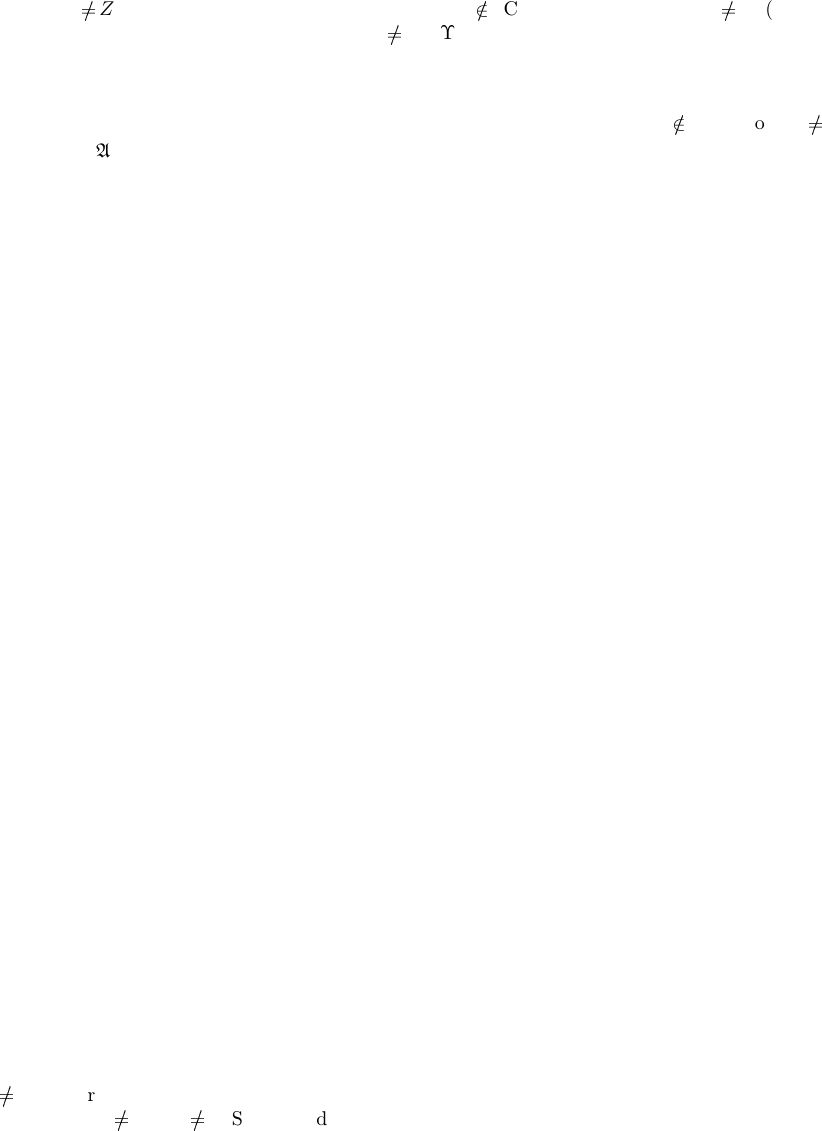2. g f
1
|
(hf i{xX
A
dom A
| ρ
A
x=A})\{Z
B
f
A)}
is injective.
Really,
1. Let y
Z
B
f
A) for some y dom ϕ
A
. Then f
1
y
ZC
A
because otherwise fx
Z
B
f
A)
for some x ZC
A
. Consequently g f
1
y
Z
C
g
A).
2. f
1
|
hf i{xX
A
dom A
| ρ
A
x=A}\{Z
B
f
A)}
is obviously injective.
g|
hf
1
i((hf i{xX
A
dom A
| ρ
A
x=A})\{Z
B
f
A)})
is injective because f
1
y
ZC
A
for y
Z
B
f
A).
Thus g f
1
|
(hf i{xX
A
dom A
| ρ
A
x=A})\{Z
B
f
A)}
is injective.
As shown by the below theorems, every two quasi-cartesian functions are equivalent up to a
bijection:
Theorem 19. ϕ
A
f |
{xX
A
dom A
| ρ
A
x=A}
=g|
{xX
A
dom A
| ρ
A
x=A}
.
Proof. If x {x X
A
dom A
| ρ
A
x = A} \ ZC
A
then
[TODO: Make clear that mult ivalued functions
are not applied below. Rewrite the proof for clarity.]
ϕ
A
f |
{xX
A
dom A
| ρ
A
x=A}
x =
gid
{xX
A
dom A
| ρ
A
x=A}
f
1
f |
{xX
A
dom A
| ρ
A
x=A}
x =
g id
{xX
A
dom A
| ρ
A
x=A}
x =
gx =
g|
{xX
A
dom A
| ρ
A
x=A}
x.
If x {x X
A
dom A
| ρ
A
x = A} ZC
A
then
f |
{xX
A
dom A
| ρ
A
x=A}
x = Z
B
Υ
f
A and
g|
{xX
A
dom A
| ρ
A
x=A}
x = Z
C
Υ
g
A;
f
1
f |
{xX
A
dom A
| ρ
A
x=A}

{x} = {x X
A
dom A
| ρ
A
x = A} ZC
A
. Thus it is easy to show that
g id
{xX
A
dom A
| ρ
A
x=A}
f
1
f |
{xX
A
dom A
| ρ
A
x=A}

{x} = {Z
C
Υ
g
A}.
Now let also f and g be with injective aggregation.
Let Φ = g f
1
.
Lemma 20. The set of all hf i{x X
0
dom A
| ρ
0
x = A}, for A being small indexed families of
forms, is a partition of the set im f where f is a quasi-cartesian function w ith injective aggregation
S
1
S
2
.
Proof. Let denote this set S. That
S
S = im f is obvious.
Suppose A = hf i{x X
0
dom A
| ρ
0
x = A
0
} and B = hf i{x X
0
dom A
| ρ
0
x = A
1
} for families
A
0
A
1
of forms. Then for every a A we have a = fx w he re ρ
0
x = A
0
. Thus ρ
1
a = ΥA
0
and
ρ
1
b = ΥA
1
; ρ
1
a
ρ
1
b; a
b. So S is a disjoint set.
Theorem 21. Φ is a bijection im f im g.
Proof. From the lemma.
4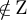Theorem 22. Φ f = g.
Proof. Because Φ f |
hf i{xX
A
dom A
| ρ
A
x=A}
=g |
hf i{xX
A
dom A
| ρ
A
x=A}
and the lemma.
Theorem 23.
1. ϕ
A
= Φ|
hf i{xX
A
dom A
| ρ
A
x=A}
for every small indexed fa m ily A of forms.
2. Φ is the union of all functions ϕ
A
where A is a small indexed family of forms.
Proof. Both are trivial from the above.
Deﬁnition 24. A product-projection system is a quasi-cartesian function together with a function
Pr whose values are indexed families, such that for every x dom f:
x
ZC
0
Pr fx = x.
[TODO: Also: f (Pr y) = y if y im f.]
[TODO: Particular product-projection systems.]
Some examples of quasi-cartesian situations and functions
Deﬁnition 25. Let C is a category with zero morphisms. The corresponding quasi-cartesian sit-
uation is:
Forms are pairs of objects.
Arguments are morphisms.
Form of an argument x is (Src x; Dst x).
Zero for form (A; B) is the zero morphism 0
AB
.
Let us prove it is really a quasi-cartesian situation.
Proof. We need to prove ρ Z ρ = ρ. Really, let f is an argument. Then ρZρf = ρZ (Src f;
Dst f) = ρ0
Src f ,Dst f
= (Src f; Dst f) = ρf.
The above deﬁnition immediately gives rise of quasi-cartesian situations for binary relations (the
category Rel), pointfree funcoids (the catego ry of small pointfree funcoids), reloids (the category
of small reloids).
Deﬁnition 26. The quasi-cartesian situation of anchored relations:
Forms F are small indexed families of sets.
Arguments are small anchored relations.
Form of an argument is the arity of anchored rel ation.
5
Zero Z for a form is the empty relation of that form.
Proposition 27. The quasi-cartesian situation of anchored relations is really a quasi-cartesian
situation.
Proof. We need to prove ρ Z ρ = ρ. Really le t f is an anchored relation of the form A. Then
Zρf is the zero relation of the same form ρf. Consequently ρZρf = ρf .
Proposition 28. Reindexation produc t (for small indexed families of relation) is a quasi-cartesian
function with injective aggregation from the quasi-cartesian situation of anchored relations to the
same quasi-cartesian situation.
Proof. First prove that it is a pre-quasi-ca rtesian function. We need to show that for every small
indexed families x, y of anchored relat ions:
1. x ZC
Q
(D)
x = Z
ρ
Q
(D)
x
;
2. ρ x = ρ y ρ
Q
(D)
x = ρ
Q
(D)
y;
that is
1. x ZC
Q
(D)
x = Z
arity
Q
(D)
x
;
2. arity x = arity y arity
Q
(D)
x = arity
Q
(D)
y;
that is
1. x ZC
Q
(D)
x = Z
arity
Q
(D)
x
;
2. arity x = arity y uncurry(arity x) = uncurry(arity y);
but these formulas are obvious.
Next prove that it is a quasi-cartesian function. We ne ed to show that for every indexed famil y of
sets
Y
(D)
x
!
|
{xX
dom A
| ρx=A}\ZC
is injection. This follows from the known fact that (
Q
x)|
{xX
dom A
| ρx=A}\ZC
is an injection.
Last, we need to pr ove that it is with injective aggregation. Deﬁne Υ(ρ x) = ρ
Q
(D)
x that is
Υ(arity x) = uncurry(arity x) that is Υ p = uncurry p. Obviously this Υ is injective.
Proposition 29. Ordinated product (for small indexed families of relation) is a quasi-cartesian
function from the quasi-cartesian situation of anchored relations to the same quasi-cartesian situ-
ation.
Proof. First prove that it is a pre-quasi-ca rtesian function. We need to show that for every small
indexed families x, y of anchored relat ions:
1. x ZC
Q
(ord)
x = Z
ρ
Q
(ord)
x
;
6
2. ρ x = ρ y ρ
Q
(ord)
x = ρ
Q
(ord)
y;
that is
1. x ZC
Q
(ord)
x = Z
arity
Q
(ord)
x
;
2. arity x = arity y arity
Q
(ord)
x = ar ity
Q
(ord)
y;
that is
1. x ZC
Q
(ord)
x = Z
arity
Q
(ord)
x
;
2. arity x = arity y
P
(arity x) =
P
(arity y);
but these formulas are obvious.
Next prove that it is a quasi-cartesian function. We ne ed to show that for every indexed famil y of
sets
Y
(D)
x
!
|
{xX
dom A
| ρx=A}\ZC
is injection. This f ollows from the known fact that (
Q
x)|
{xX
dom A
| ρx=A}\ZC
is an injection.
[TODO: More detailed proof.]
Deﬁnition 30 . The quasi-cartesian situation of pointfree funco id s over posets with least elements
is:
1. Forms are pairs (A; B) of posets with least elements.
2. Arguments are pointfree funcoids .
3. The form of an argument f is (Src f; Dst f ).
4. Zero of the form (A; B) is 0
FCD(A;B)
= (A × {0
B
}; B × {0
A
}). (It exists because A and B
have least elements.)
Proposition 31. It is really a quasi-cartesian situation.
Proof. We need to prove ρ Z ρ = ρ. Really,
ρZρf = ρZ(Src f ; Dst f) = ρ0
FCD(Src f ;Dst f)
= (Src f; Dst f) = ρf.
Deﬁnition 32. The quasi-carte s ia n situation of binary relations is:
1. Forms are pairs (A; B) of sets.
2. Arguments are Rel-morphisms;
3. The form of an argument f is (Src f; Dst f ).
4. Zero of the f orm (A; B) is the Rel-morphism (; A; B) .
7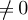Proposition 33. It is really a quasi-cartesian situation.
Proof. We need to prove ρ Z ρ = ρ. Really,
ρZρf = ρZ(Src f ; Dst f) = ρ (; Src f; Dst f) = (Src f; Dst f ) = ρf .
Deﬁnition 34. The quasi-carte s ia n situation of reloids is:
1. Forms are pairs (A; B) of sets.
2. Arguments are reloids.
3. The form of an argument f is (Src f; Dst f ).
4. Zero of the f orm (A; B) is 0
RLD(A;B)
.
Proposition 35. It is really a quasi-cartesian situation.
Proof. We need to prove ρ Z ρ = ρ. Really,
ρZρf = ρZ(Src f ; Dst f) = ρ0
RLD(Src f;Dst f)
= (Src f; Dst f ) = ρf.
Next we need to prove that cross-composition product of some p articular categories with star-
morphisms are quasi-cartesian functions with injective aggregation.
Theorem 36. Cross-composition product (for small i ndexed families of relations) is a quasi-carte-
sian function with injecti ve aggregation from the quasi-cartesian situation S
0
of binary relations
to the quasi-cartesian situation S
1
of pointfree funcoids over posets with least elements.
Proof. First prove that it is a pre-quasi-ca rtesian function. We need to show that for every small
indexed families x, y of Rel-morphisms:
1. x ZC
0
Q
(C)
x = Z
1
ρ
1
Q
(C)
x
;
2. ρ
0
x = ρ
0
y ρ
1
Q
(C)
x = ρ
1
Q
(C)
y;
Q
(C)
x = Z
1
ρ
1
Q
(C)
x
Q
(C)
x = Z
1
(FCD(StarHom(λi dom x: Src x
i
); StarHom(λi dom x:
Dst x
i
)))
Q
(C)
x = 0
FCD(StarHom(λidom x:Src x
i
);StarHom(λidom x:Dst x
i
))
a StarHom(λi
dom x: S rc x
i
):
D
Q
(C)
x
E
a = 0
StarHom(λidom x :Dst x
i
)
a StarHom(λi dom x: Src x
i
):
StarComp(a; x) = 0
StarHom(λidom x :Dst x
i
)
a StarHom(λi dom x: Src x
i
): GR StarComp(a;
x) = ;
a StarHom(λi dom x: Src x
i
): GR StarComp(a; x) = x Z C
0
.
a StarHom(λi dom x: Src x
i
): GR StarComp(a; x) = GR StarComp
dom x;
Q
idom x
Src x
i
; x
= L
arity a
y
Q
idom x
Src x
i
i arity a: y
i
x
i
L
i
¬∀i arity aL , y Src x
i
: yx
i
L ¬∀i a rity a: x
i
0 x ZC
0
.
Thus x ZC
0
Q
(C)
x = Z
1
ρ
1
Q
(C)
x
.
If ρ
0
x = ρ
0
y then arity x = arity y = n for some index set n.
8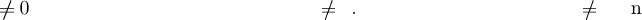ρ
0
x = ρ
0
y λi n: (Src x = Src y Dst x = Dst y) ρ
1
Q
(C)
x = FCD(StarHom(λi dom x:
Src x
i
); StarHom(λi dom x: Dst x
i
)) = FCD(StarHom(λi dom y: Src y
i
); StarHom(λi dom y:
Dst y
i
)) = ρ
1
Q
(C)
y.
We have proved that it is a pre-quasi-cartesian fun ction.
Next prove that it is a quasi-ca rtesian function, that is
Y
(C)
!
|
{xX
0
dom A
| ρ
0
x=A}\ZC
0
is an injection for every indexed family A of forms. Let x {x X
0
dom A
| ρ
0
x = A} \ ZC
0
. To
prove that it is an injection we will restore the value of x from
Q
(C)
x.
D
Q
(C)
x
E
{p} = StarComp({p}; x) for every p
n
.
L GR Sta rComp({p}; x) i n: p
i
x
i
L
i
i n: L
i
hx
i
i{p
i
} for every L
n
.
Thus GR StarComp({p}; x) =
Q
in
hx
i
i{p
i
}.
Since x
i
0 there exist p such that hx
i
i{p
i
}
0. Take k n, p
i
= p
i
for i
k an d p
k
= q for an
arbitrary value q; then
hx
k
i{q } = Pr
k
Y
in
hx
i
i{p
i
} = Pr
k
GR StarComp({p
}; x) = Pr
k
GR
*
Y
(C)
x
+
{p
}.
So the value of x can be restored from
Q
(C)
x by this formula.
It remained to prove that it is with injective aggregat ion.
We have ΥF = (Sta rHom(λi dom f: F
i,0
); StarHom(λi dom f : F
i,1
)) for every form F .
It is really an injection because Star Hom() are disjoint.
Theorem 37. Cross-composition product (for small indexed families of pointfree funcoids between
separable atomic poset s with leas t elements and atomistic posets) is a quasi-cartesian function
(with injective aggregation) from the quasi-cartesian situation S
0
of pointfree funcoids over posets
with least elem ents to the q uasi-cartesian situation S
1
of pointfree funcoids over posets with least
elements.
Proof. First prove that it is a pre-quasi-ca rtesian function. We need to show that for every small
indexed families x, y of pointfree funcoids:
1. x ZC
0
Q
(C)
x = Z
1
ρ
1
Q
(C)
x
;
2. ρ
0
x = ρ
0
y ρ
1
Q
(C)
x = ρ
1
Q
(C)
y;
Q
(C)
x = Z
1
ρ
1
Q
(C)
x
Q
(C)
x = Z
1
(FCD(StarHom(λi dom x: Src x
i
); StarHom(λi dom x:
Dst x
i
)))
Q
(C)
x = 0
FCD(StarHom(λidom x:Src x
i
);StarHom(λidom x:Dst x
i
))
a StarHom(λi
dom x: S rc x
i
):
D
Q
(C)
x
E
a = 0
StarHom(λidom x :Dst x
i
)
a StarHom(λi dom x: Src x
i
):
StarComp(a; x) = 0
StarHom(λidom x :Dst x
i
)
a StarHom(λi dom x: Src x
i
): GR StarComp(a;
x) = ;
a StarHom(λi dom x: Src x
i
): GR StarComp(a; x) = x Z C
0
.
9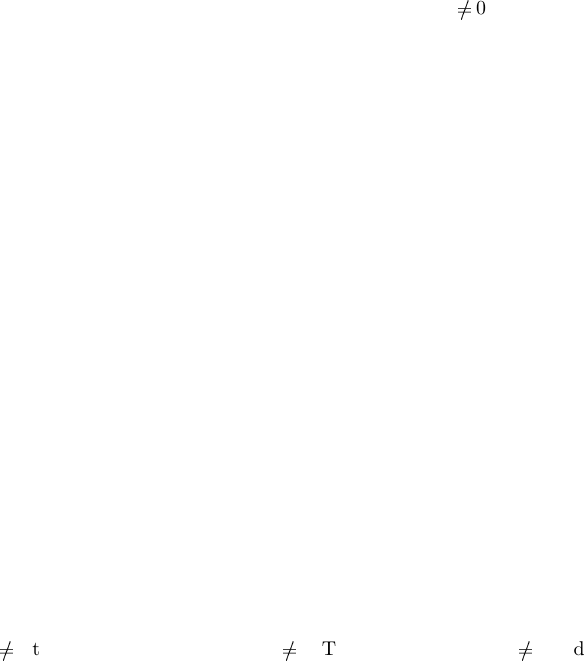a StarHom(λi dom x: Src x
i
): GR StarComp(a; x) = GR StarComp
dom x;
Q
idom x
Src x
i
; x
= L
arity a
y
Q
idom x
Src x
i
Q
idom x
atoms Src x
i
i
arity a: y
i
[x
i
] L
i
L
arity a
y
Q
idom x
atoms Src x
i
i arity a: y
i
[x
i
] L
i
¬
i arity aL , y atoms Src x
i
: y [x
i
] L ¬∀i arity a: x
i
0 x ZC
0
.
Thus x ZC
0
Q
(C)
x = Z
1
ρ
1
Q
(C)
x
.
If ρ
0
x = ρ
0
y then arity x = arity y = n for some index set n.
ρ
0
x = ρ
0
y λi n: (Src x = Src y Dst x = Dst y) ρ
1
Q
(C)
x = FCD(StarHom(λi dom x:
Src x
i
); StarHom(λi dom x: Dst x
i
)) = FCD(StarHom(λi dom y: Src y
i
); StarHom(λi dom y:
Dst y
i
)) = ρ
1
Q
(C)
y.
We have proved that it is a pre-quasi-cartesian fun ction.
Next prove that it is a quasi-ca rtesian function, that is
Y
(C)
!
|
{xX
0
dom A
| ρ
0
x=A}\ZC
0
is an injection for every indexed family A of forms. Let x {x X
0
dom A
| ρ
0
x = A} \ ZC
0
. To
prove that it is an injection we will restore the value of x from
Q
(C)
x.
D
Q
(C)
x
E
p = StarComp(p; x) for every p
Q
in
atoms Src x
i
.
It is easy to see that GR p
Q
in
atoms Src x
i
= {p}. Thus
L GR Sta rComp(p; x) i n: p
i
[x
i
] L
i
i n: L
i
hx
i
ip
i
for every L
Q
in
Src x
i
.
Thus GR StarComp(p ; x) =
Q
in
hx
i
ip
i
.
Since x
i
0 there exist p such that hx
i
ip
i
0. Take k n, p
i
= p
i
for i
k and p
k
= q fo r an arbitrary
value q; then
hx
k
iq = Pr
k
Y
in
hx
i
ip
i
= Pr
k
GR StarComp(p
; x) = Pr
k
GR
*
Y
(C)
x
+
p
. (2)
Note that the theorem ?? in [
?] applies to every x
i
.
So the value of x can be restored from
Q
(C)
x by this formula.
It remained to prove that it is with injective aggregat ion.
We have ΥF = (Sta rHom(λi dom f: F
i,0
); StarHom(λi dom f : F
i,1
)) for every form F .
It is really an injection because Star Hom() are disjoint.
Conjecture 38. Cross-composition produ ct (for small indexed families of reloids) is a quasi-
cartesian function (with injective aggregation) from the quasi-cartesian situation S
0
of reloids to
the quasi-cartesian situation S
1
of pointfree funcoids over posets with least elements.
Remark 39. The above conjecture is unsolved even for pr oduct of two multipliers.
Theorem 40. Reloidal product (for small indexed families of ﬁlters on powersets) with multireloid
projections is a product-projection system with injective aggregation from the quasi-cartesian situ-
ation S
0
of ﬁlte rs to the quasi-cartesian situation S
1
of multireloids.
10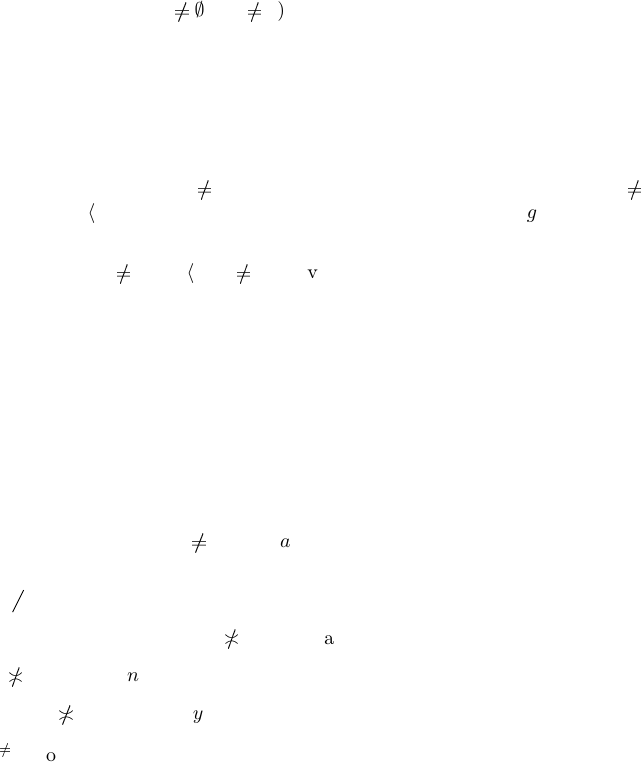Ordered quasi-cartesian situations
Deﬁnition 41 . An ordered quasi-cartesian situation is a quasi-cartesian situation together with a
partial order on each its set of its arguments of each given form.
Deﬁnition 42. An order-p reserving quasi-cartesian function from a quasi-cartesian situ at io n S
0
to a quasi-ca rtesian situation S
1
is a quasi-cartesian function σ such that σx σy x y for
every indexed family A of forms and x, y {x X
0
dom A
| ρ
0
x = A} \ ZC
0
.
Obvious 43. Every order-preserving quasi-cartesian function is a quas i-cartesian function with
injective aggregatio n.
Remark 4 4. Using the obvious fact above, we can prove again that the considered quasi-cartesian
functions are with injective aggregation using the below proved statements that they are order-
preserving.
Proposition 45. Cross-composition product (for small indexed families of relations) is an order-
preserving quasi-cartesian function from the quasi-cartesian situation S
0
of binary relations to the
quasi-cartesian situation S
1
of pointfree funcoids over posets with least elements equipped with the
usual orderings of these sets.
Proof. We nee d to prove i n: (f
i
g
i
)
Q
C
f
Q
C
g f g for every n-indexed
families f and g of binary relations.
D
Q
(C)
f
E
Q
a =
Q
in
hf
i
ia
i
.
Fix k n, x . Let a =
n\{k}
{(k; x)}. Then
*
Y
(C)
f
+
Y
a =
Y
in
hf
i
i if i
k;
hf
k
i{x} if i = k.
and
*
Y
(C)
g
+
Y
a =
Y
in
hg
i
i if i
k;
hg
k
i{x} if i = k.
Taking into account that hf
i
i
and hg
i
i
for every i n, by properties of Cartesian product,
we get hf
k
i{x} hg
k
i{x} for every x and thus f
k
g
k
.
Corollary 46. Cross-composition product (for small indexed families of Rel -morphisms) is an
order-preserving quasi-cartesian function from the quasi-cartesian situation S
0
of Rel- m orphisms
to the quasi-cartesian situation S
1
of pointfree funcoids over posets with least elements.
Theorem 47. Let each A
i
(for i n where n is s ome index set) is a separable poset with least
element. Then
i n: a
i
0
Y
FCD
a
Y
FCD
b a b.
Proof. Suppose a
b.
Q
A is a separable poset, Thus it exists y
a such that y b.
We have i n: y
i
a
i
and i n: y
i
b
i
.
Take k n such that y
k
a
k
. We have y
k
b
k
.
Take z
i
=
a
i
if i
k;
y
k
if i = k
for i n.
11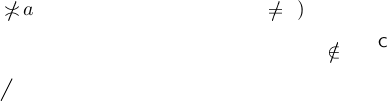i n: z
i
a
i
(taken in account tha t a
i
0) and i n : z
i
b
i
.
So there exists z such that z
Q
FCD
a and z
Q
FCD
b.
Q
FCD
a
Q
FCD
b.
Corollary 48.
Q
FCD
is an order-preserving quasi-cartesian function from the (deﬁned in an
obvious way) quasi-cartesian situation of separable posets with least elements to the (deﬁned in an
obvious way) quasi-cartesian situation of multifuncoids.
[TODO: Write the deﬁnitions explicitly.]
Theorem 49. Cross-composition product (for small indexed families of pointfree funcoids between
separable atom ic posets with least e lements and atomistic posets) is an order-preserving quasi-
cartesian function from the quasi-cartesian situation S
0
of pointfree funcoids over posets with least
elements to the quasi-cartesian situation S
1
of pointfree funcoid s over posets with least elements.
Proof. It follows from the formula (2).
[TODO: More detailed proof.]
[TODO: Ordinated product is a quasi-cartesian function with injective aggreg ation.]
[TODO: Reloidal product is an order-preserving quasi-cartesi an function.]